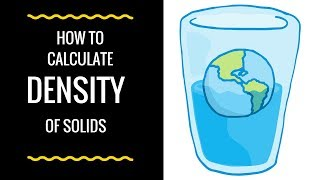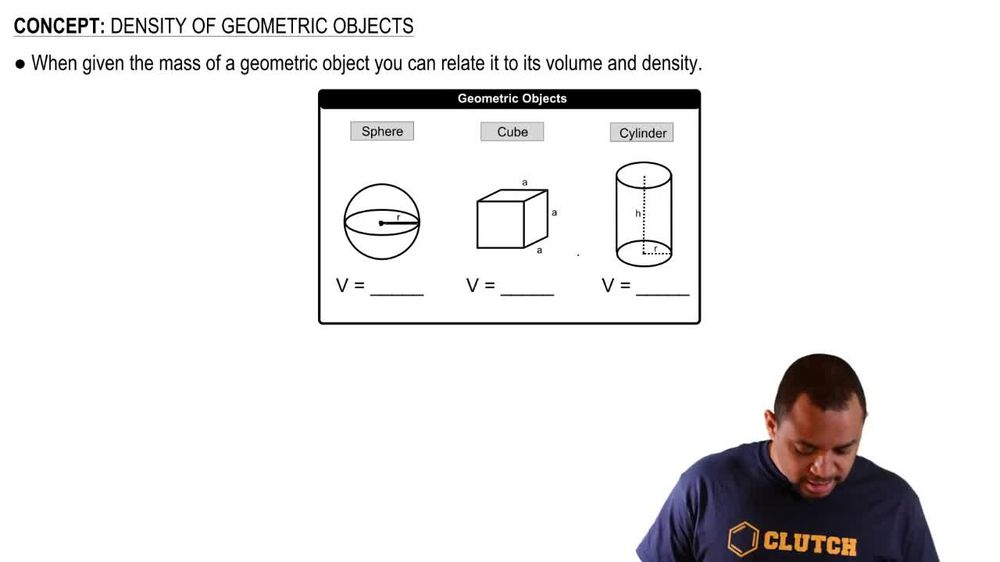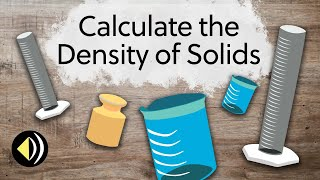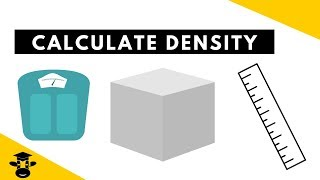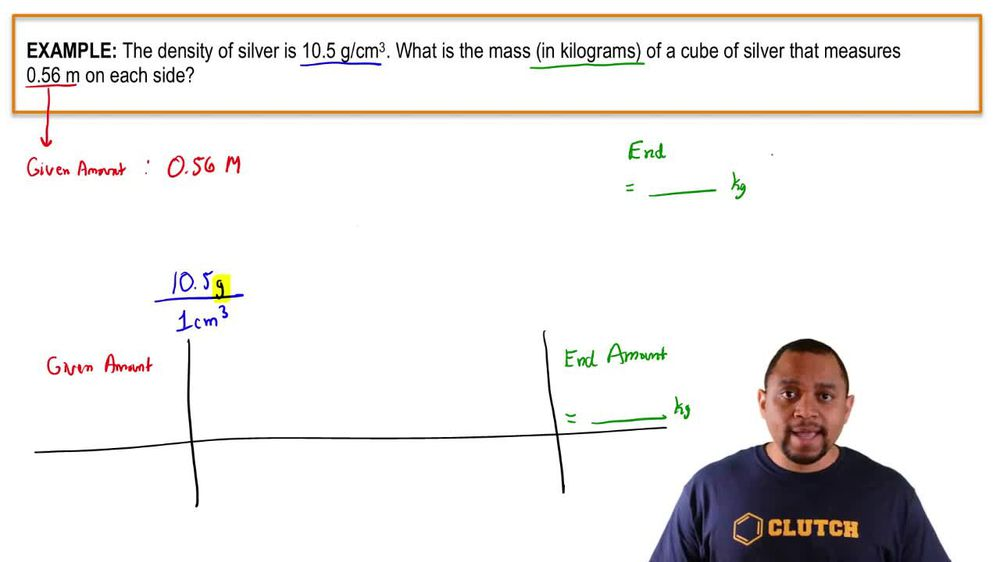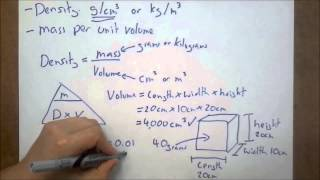Start typing, then use the up and down arrows to select an option from the list.
1. 1. Intro to General Chemistry2. Density of Geometric Objects
Problem

# Brass is a copper–zinc alloy. What is the mass in grams of a brass cylinder having a length of 1.62 in. and a diameter of 0.514 in. if the composition of the brass is 67.0% copper and 33.0% zinc by mass? The density of copper is 8.92 g/cm3, and the density of zinc is 7.14 g/cm3. Assume that the den-sity of the brass varies linearly with composition.

Relevant Solution4m
Play a video: